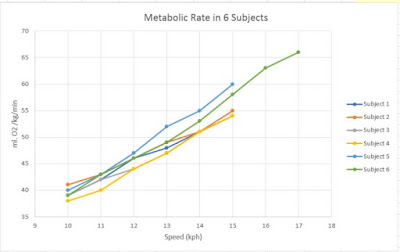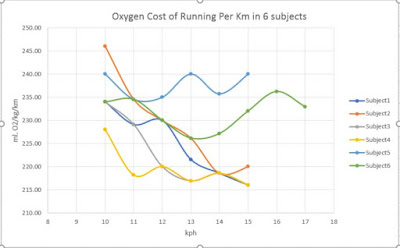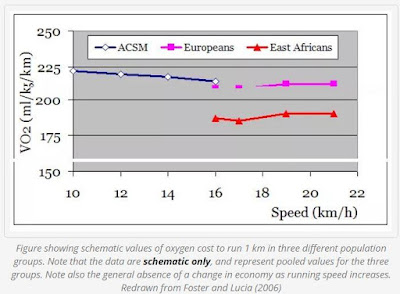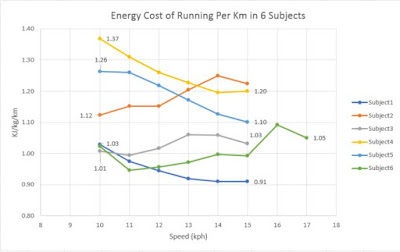## Monday, December 24, 2018

### Surface Related VO2 Changes Not Reflected In Stryd Power - An Examination of Aubrey et.al

In a recent paper by Aubrey et.al published in the Journal of Strength and Conditioning Research, significant differences in oxygen consumption were reported from treadmill to overground running without evidence of a corresponding change in Stryd reported running power. Details can be found here

Two quick pieces of summary :

a) The main bit of detail is that there was a significant change in VO2 not reflected in corresponding power readings between treadmill and overground running.

b) The other statement made in the paper is that a weak correlation was found between oxygen cost and power:weight ratio across all runners, elite or recreational, suggesting that "running power as assessed with the Stryd Power Meter, is not a great reflection of the metabolic demand of running in a mixed ability population of runners".

In a rebuttal of point b) in the paper, Dr. Snyder from Stryd accused the authors of "fatal methodological flaws" when they chose to normalize both metabolic rate and power/weight ratio with speed while pointing to a weak correlation between the two variables (r = 0.29, p = 0.02).

Dr. Snyder's rebuttals are examined with the help of data from our old friends, Dutch researchers from the Secret of Running group. From their blog, I extracted mean VO2 and mean power/weight ratios from treadmill testing belonging to a subset of 6 runners in random fashion.

Statement 1 : Rate of oxygen consumption is approximately proportional to speed (linearly dependent upon speed with a y-intercept of close to zero) across both elite and recreational runners (Batliner et al., 2018). This means all values for the rate of oxygen consumption measure when normalized by speed (otherwise known as cost of transport)* will be approximately constant, giving virtually no variation in these values other than that due to noise or subject variation. Therefore, regardless of Stryd power’s dependence upon speed, no correlation would be expected between the normalized measures.

Aubrey et.al normalized metabolic rate in ml/kg/min with speed measured in m/s. Such a division does not result automatically in the oxygen cost of transport.

Infact, the actual formula is :

Oxygen cost = 60/3.6*VO(ml O2/kg/min)/v (m/s)  --- 1)

So by calling this normalization "cost of transport", Dr. Snyder is not dimensionally correct because the x-axis in the Aubrey paper shows values ranging from 9 to 17 (see Figure 1 in their paper). Such low double digit values cannot align with the oxygen cost of running, which is in the triple digits.

Behavior of oxygen consumption with speed can be examined from the data of Secret of Running. The plot in Fig.1 shows that for 6 different subjects, metabolic rate is mostly linearly proportional to speed.Fig 1 : Metabolic rate vs running speed measured in 6 subjects. Source of data : Secret of Running (Dijk, Megen).

Converting these values to an oxygen cost of running with the appropriate formula in 1) transforms the plot into the following plot in Fig.2. As Dr. Snyder states, the linear relationship between speed and oxygen consumption becomes nearly constant save for noise and subject variation. Infact, when looking at this plot, the data looks less noisy for some runners (4,5,6) and more noisy for others (1,2,3). What is the source of this variation? Some explanation would be good.Fig 2 : Oxygen cost of running vs running speed in 6 subjects. Source of data : Secret of Running (Dijk, Megen).

What does research say about this relationship? According to the plot in Fig.3, there is a "general absence" of a change in oxygen cost as running speed increases.  However, because of the noise from the Stryd sensor, this constant relationship is not exactly seen.

From looking at Fig.2, we cannot make the claim that some individuals somehow magically reduce their oxygen cost as speed increases. The fundamental source of these fluctuations appear to be noise. It is precisely this noisy bit that requires further examination if such devices are to be applied among elite runners as a "surrogate" measure of oxygen cost. Not that I didn't warn about it on the Stryd Facebook page many moons agoFig 3 : Oxygen cost in three different population groups

Statement 2 : Stryd power’s strong linear correlation with rate of oxygen consumption, however, indicates increasing Stryd power with increasing speed, meaning any variability would be reduced by normalization with speed. Thus, any correlation whatsoever between the normalized measures would be small and due to chance, unaccounted for nonlinearities, or subject variation, not the dominant linear relation with speed that underlies both non-normalized measures.

The relationship between Stryd power/weight ratio and treadmill speed can be examined in the Secret of Running data. By way of algorithmic implementation, Stryd power/weight in strongly linear in speed (Fig.4). But on closer inspection, not all subjects show linear proportionality. Infact, in this data, there doesn't appear to be anything close to perfectly linear relationship. Almost all datapoints show a wavy pattern.

Some appear comical. Subject 6 shows markedly high power ramp beween 15 and 16 kph compared to that between 16 and 17kph.

Subject 1 on the other hand exhibits something that looks like a curvilinear relationship.

What is the cause of these artifacts?

There is no reason why some runners should take more effort to "jump" between two speeds compared to other speeds. The treadmill test is a continuously administered test with no "breaks" in between each speed. The other explanation could be the choice of value of VO2. It hasn't been explained by the authors of Secret of Running on what basis they chose steady state values. Were some intervals shorter than others, affecting the average of VO2 in that interval?Fig 4 : Stryd power/weight ratio vs running speed in 6 subjects. Source of data : Secret of Running (Dijk, Megen).
Normalizing the power/weight values by speed will dimensionally yield the energy cost of running through the formula :

ECOR (kJ/kg/km) = P (Watt/kg)/v (m/s)  ---- 2)

When the above data is normalized by speed using the expression in 2), we get the following plot. Again, due to random variations in the Stryd data, none of the subjects show a constancy in energy cost of running.Fig. 5 : ECOR (calculated) in 6 subjects. Source of data : Secret of Running (Dijk, Megen).

The authors in Secret of Running have argued that the differences in ECOR among runners is of a fundamental nature due to some being more experienced and more "efficient" than others. They suggest in their literature and books that it is important to reduce ECOR and that the Stryd powermeter is sensitive enough to measure ECOR.

However, I challenge this idea. I suggest that these authors re-examine if changes in ECOR are really due to training status and running experience or simply due to random variations in the data as Fig. 5 and Dr. Snyder's assertion shows! Otherwise, different interpretations from different people appear to conflict.

Statement(s) 3 : [...] there is still a very strong linear relationship between the rate of oxygen consumption values and the Stryd power values. This strong dependence is obviously significantly reduced when these values are normalized by speed, giving a value only slightly larger than that found in the paper.
[....]Stryd power data are tailored to the individual, with power calculations being performed using input data for each specific subject, not across subjects. Therefore, if one were to actually validate Stryd power’s values as a training metric, as the paper’s title implies, correlation coefficients between rate of oxygen consumption and Stryd power should only be performed on a subject-by-subject basis

In keeping with Dr. Snyder's advice of analying Stryd data strictly on a subject-by-subject basis, I plot W/kg and metabolic rate of individual subjects separately on one plot and examine the strength of trendline linearity (Fig 6). Each subject's trendline and co-efficient of determination is shown. The plot shows that changes in metabolic rate explain anywhere from 96% to 98% of the variation in W/kg. The relationship is strong but far from 100%. It also shows a similar picture to the data I have collected from my own laboratory VO2max testingFig. 5 : Energy cost lof running (calculated) vs cxygen cost of running (calculated).  Source of data : Secret of Running (Dijk, Megen).

What Aubrey et.al did in their paper (Figure 1) was pool all runner's data together by normalizing the metabolic rate and power/weight ratio by running speed. If we do the same for dataset from Secret of Running, all relationships are blunted and the plot essentially becomes a scatter of points (Fig.6).Fig 6 : Normalized specific power vs normalized VO2.  Source of data : Secret of Running (Dijk, Megen).

So the methodological error explained by Dr. Snyder seems to be correct. Aubrey et.al must explain why they took this approach and on which former pieces of literature they borrowed this kind of analysis.

Stryd power and VO2 show a significant linear relationship. This relationship is pegged in two ways.

1) The Stryd powermeter, by way of algorithm, reports increased watts with increased running speed on flat surfaces.

2) By VO2 being positively proportional to speed on flat land running.

Statement 4 : Data collection methods are not consistent across surfaces, making effective comparison across surfaces impossible.

That Aubrey et.al didn't fully explain data collection methods is a genuine accusation. However, on the same token, articles published by Secret of Running that were used in the chain emailing marketing efforts by Stryd also lacked tremendous clarity on how the authors conducted the tests.

For example, the authors Dijk & Megen stated that the energy cost of running increases uphill. The exact magnitude of the increase is in question. Is the nature of the specific increase just due to how the numerator in the algorithm (W/kg) is scaled to increase faster than the denominator (speed) and on what basis were the scaling factors decided?  The correlational aspects of Stryd power and above ground gradient running is left to be explored and explained in scientific literature.

Conclusion

The methodological "fatal flaw" explained by Dr. Snyder in the Aubrey paper seems to be correct. Aubrey et.al must explain why they took this approach and on which former pieces of literature they borrowed this kind of analysis from. A proper explanation for this choice is desired.

On cross-examining statements made with other data from Secret of Running group, Stryd power to weight ratio has a significant positively proportional relationship with speed. However, the data is not exactly linear, more wavy due to the presence of random variations and subject related issues and the slope of a linear trend line varies with subject.

Both the energy cost of running and the oxygen cost of running calculated by normalizing power/weight ratio and metabolic rate by speed respectively are not exactly constant when seen in practice. Constancy is shown in literature but real data appears wavy, sometimes monotonically decreasing in certain runners. This maybe due to random errors in the sensor  and variations in sensor placement as well as experimental issues in the VO2 data but these facts needs to be appreciated.

Therefore, the Stryd as a powermeter must be used to make assertions about metabolic fitness only within subjects, as oppoed to across subjects.

If we assume for a moment that Aubrey et.al indeed did due diligence and considered steady state VO2 values across both treadmill and above ground running, the Stryd research team has left some explaining to do why the observed differences in oxygen cost did not reflect in a corresponding difference in Stryd power. At the heart of this explanation lies several extrapolations various people are making on the internet about energy cost of running, running efficiency and oxygen economy, all on the basis of algorithms and no direct measurements of force or power.